# Tangent and Normal JEE Notes | EduRev

## JEE : Tangent and Normal JEE Notes | EduRev

The document Tangent and Normal JEE Notes | EduRev is a part of the JEE Course Mathematics (Maths) Class 12.
All you need of JEE at this link: JEE

A. Tangent & Normal

Definition : The tangent line to the graph of f at the point P(a, f(a)) is

(1) the line on P with slope f'(a) if f'(a) exists ;

(2) the line x = a if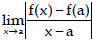= ∞.

In neither (1) nor (2) holds, then the graph of does not have a tangent line at the point P(a, f(a)).

In case f'(a) exists, then y - f(a) = f'(a) (x - a)

is an equation of the tangent line to the graph of f at the point P(a, f(a)).

The normal line N to the graph of a function f at the point P(a, f(a)) is defined to be the line through P perpendicular to the tangent line.

It follows that if f'(a)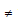0 the slope of N is -1/f'(a) and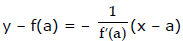is an equation of N.

If f'(a) = 0, then N is the vertical line x = a; and if the tangent line is vertical, then N is the horizontal line y = f(a).

Note :

1. The point P (x1 , y1) will satisfy the equation of the curve & the equation of tangent & normal line.

2. If the tangent at any point P on the curve is parallel to the axis of x  then  dy/dx = 0 at the point P.

3. If the tangent at any point on the curve is parallel to the axis of y, then dy/dx = ∞ or dx/dy = 0.

4. If the tangent at any point on the curve is equally inclined to both the axes there dy/dx = ± 1.

5. For equation of tangent at (x1, y1), substitute xx1 for x2, yy1 for y2,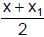for x,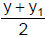for y and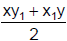for xy and keep the constant as such. This method is applicable only for second degree curves, i.e., ax2 + 2hxy + by2 + 2gx + 2fy + c = 0

6. Method to find normal at (x1, y1) of second degree conics ax2 + 2hxy + by2 + 2gx + 2fy + c = 0 The equation of normal at (x1, y1) is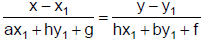Ex.1 Find the equation of tangent to the ellipse 3x2+y2+x+2y = 0 which are perpendicular to the line 4x - 2y = 1.

Sol. Since, tangent is the perpendicular to the line 4x - 2y = 1,

(slope of tangent) × (slope of normal) = –1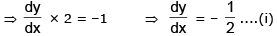The given equation 3x2 + y2 + x + 2y = 0 ....(iii)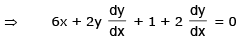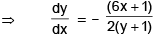Let (x1, y1) be the point of contact of the tangent and the curve

From (i) and (iii), we get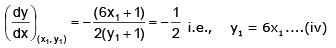Substituting this in (ii) [since the points lies on the curve] we get,

3x12 + 36x12 + x1 + 12x1 = 0

i.e., 13x(3x1 + 1) = 0 ⇒ x1 = 0, –1/3

Using (iv), x= 0 ⇒ y1 = 0 and x1 = –1/3 ⇒ y1 = –2

Hence, the points where tangent has slope –1/2 are P(0, 0) and Q(–1/3, –2).

Equation of tangents at P, Q are y  = -1/2x i.e. x+2y = 0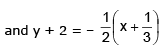i.e., 3x + 6y + 13 = 0 respectively..

Ex.2 Find the equation of normal to the curve x + y = xy, where it cuts x-axis.

Sol.

Given curve is x + y = xy .....(i)

at x–axis y = 0,  x + 0 = x0 ⇒ x = 1

Point is A(1, 0)

Now to differentiation x + y = xy taking log of both sides

⇒ log(x + y) = y log x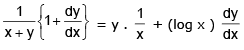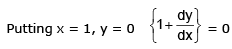⇒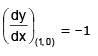slope of normal = 1

Equation of normal is,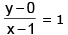⇒ y = x – 1

Ex.3 At what points on the curve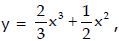the tangents make equal angles with co-ordintae axes ?

Sol.

Given curve is....(1)

Differentiating both sides w.r.t.x, then dy/dx = 2x2 + 2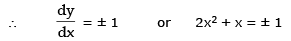or 2x2 + 2x – x – 1 = 0  or  (2x – 1) (x + 1)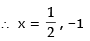(If 2x+x + 1 = 0 then x is imaginary)

From (1), for x = 1/2,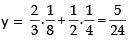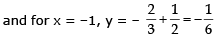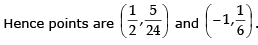Ex.4 Show that the curve x = 1 - 3t2, y = t - 3t3 is symmetrical about x-axis and has no real point for x > 1. If the tangent at the point t is inclined at an angle φ to OX. Prove that 3t = tanφ + secφ. If the tangent at P(-2, 2) meets the curve again at Q, prove that the tangents at P and Q are at right angles.

Sol. Given curve is x = 1 - 3t2  ...(1)

&  y = t - 3t3    ...(2)

From (1) and (2), y = tx   or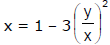⇒  x3 = x2 - 3y

Since all powers of y are even, so curve is symmetrical about x-axis.

For x > 1 ⇒ 1 – 3t2 > 1 ⇒ –3t2 > 0 Impossible

From (1) and (2),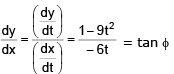...given (3)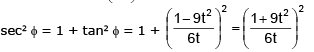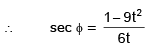Adding (3) and (4) we get, tan ø + secø = 3t

P(–2, 2)

1 – 3t2 = –2 and 2 = t – 3t3 then we get t = –1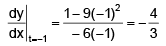Equation of tangent at (–2, 2) is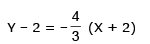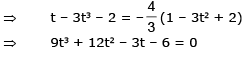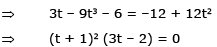Therefore the tangent at t = –1 meets the curve again at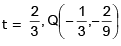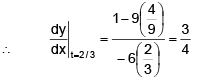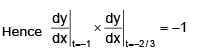Hence the tangents at P and Q are at right angles.

Ex.5 Tangent at P(2, 8) on the curve y = x3 meets the curve again at Q. Find coordinates of Q.

Sol. Equation of tangent at (2, 8) is y = 12x - 16

Solving this with y = x3 we get x3 - 12x + 16 = 0

this cubic must give all points of intersection of line and curve y = x3

i.e., point P and Q.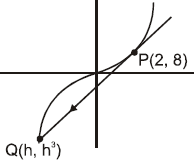But, since line is tangent at P so x = 2 will be a repeated root of equation x3 -12x + 16 = 0 and another root will be x = h.

Using theory of equations sum of roots ⇒ 2 + 2 + h = 0   ⇒ h = -4

Hence coordinates of Q are (-4, -64)

Ex.6 If the normal to the curve x2/3 + y2/3 = a2/3 makes an angle φ with the axis of x, show that its equation is y cosφ - x sinφ = a cos 2φ.

Sol. Given curve is x2/3 + y2/3 = a2/3 ...(1)

Differentiating both sides w.r.t.x, we get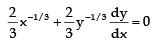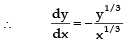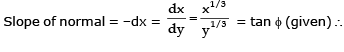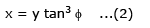From (1) and (2),  y2/3 (1 + tan2Ø) = a2/3 Þ  y2/3 = a2/3 cos2Ø

y = a cos3 Ø and x = a sin3 Ø

Therefore equation of normal is y – a cos3 Ø = tan Ø (x – a sin3 Ø)

y cos Ø– a cos4 Ø = x sin Ø – a sin4 Ø

y cosØ– x sin Ø = a (cos4 Ø– sin4 Ø)

= a (cos2 Ø + sin2 Ø) (cos2 Ø- sin2 Ø) = a . 1 . cos 2Ø

Hence y cos Ø– x sin Ø = a  cos 2Ø

Ex.7 (a) Find y' if x3 + y3 = 6xy.

(b) Find the tangent to the folium of Descartes x3 + y3 = 6xy at the point (3, 3).

(c) At what points on the curve is the tangent line horizontal ?

Sol. (a) Differentiating both sides x3 + y3 = 6xy with respect to x, regarding y as a function of x, and using the Chain Rule on the y3 term and the Product Rule on the 6xy term, we get

3x+ 3y2y' = 6y + 6xy' or x2 + y2y' = 2y + 2xy'

We now solve for y' :y2y' – 2xy' = 2y – x2

(y– 2x)y' = 2y – x2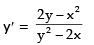(b) when x = y = 3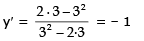So, tangent to the folium of Descarte is y – 3 = –1(x – 3)    or x + y = 6

(c) The tangent line is horizontal if y' = 0. Using the expression for y' from part (a), we see that y' = 0 when 2y – x2 = 0. Substituting y = 1/2x2 , n the equation of the curve, we get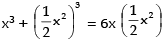which simplifies to x6 = 16x3. so either x = 0 or x= 16. If x = 161/3 = 24/3, then y = 1/2(28/3) = 25/3. Thus, the tangent is horizontal at (0, 0) and at (24/3, 25/3).

Ex.8 In the curve xa yb = ka + b, (a b > 0) prove that the portion of the tangent intercepted between the coordinate axes is divided at its point of contact into segments which are in constant ratio.

Sol. Let P(x1, y1) be the point of contact of the tangent.

Here, xayb = ka + b

∴ a log x + b log y = (a + b) log k.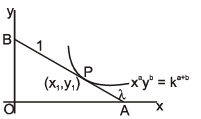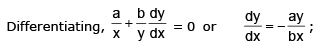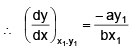Solving with y = 0,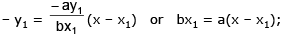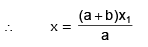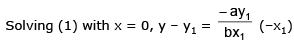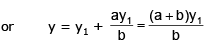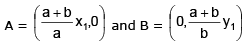Let P divide AB in the ratio λ : 1. Then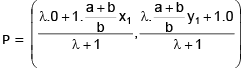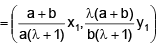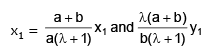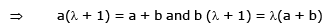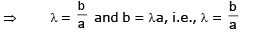P divides AB in the constant ratio b : a.

Offer running on EduRev: Apply code STAYHOME200 to get INR 200 off on our premium plan EduRev Infinity!

## Mathematics (Maths) Class 12

209 videos|222 docs|124 tests

,

,

,

,

,

,

,

,

,

,

,

,

,

,

,

,

,

,

,

,

,

;